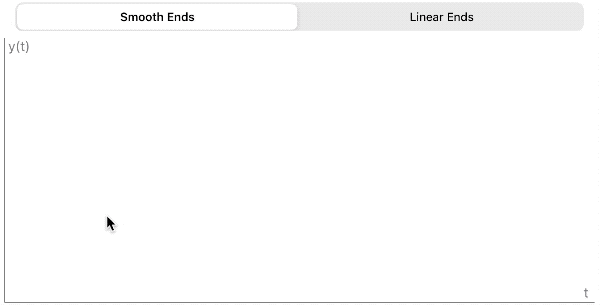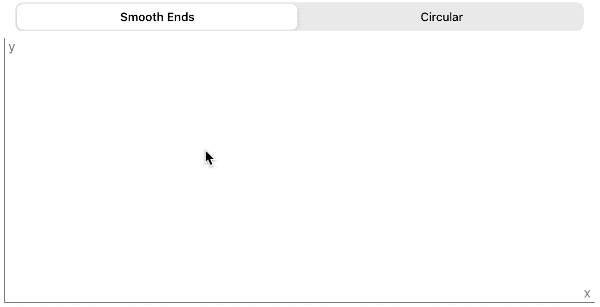Swiftpack.co -  Package - Bersaelor/SwiftSplines
Swiftpack.co is a collection of thousands of indexed Swift packages. Search packages.
Bersaelor/SwiftSplines
Piece wise spline definition for interpolation functions
.package(url: "https://github.com/Bersaelor/SwiftSplines.git", from: "0.3.0")

# SwiftSplinesI need a function that connects f(0)=0.1, f(1)=1.5 and f(2)=1 with a smooth line.

With SwiftSplines you can easily create smooth functions that interpolate control points.

Simple function (1D):2D Curve:## Features

• ☑ Interpolate points with a piecewise cubic spline
• ☑ Written for generic image space, called `DataPoint`, which can be `float`, `double`, `CGPoint` or vectors of arbitrary dimension
• ☑ Matrix calculation to get the derivatives at the control points uses Accelerate's sparse matrix solvers, so built for speed on all platforms that support `Accelerate`
• ☑ Offers smooth, fixed ends or circular boundary conditions
• ☐ use `Double`/`Float` accelerate functions depending on intput data. So far scalars are converted to `Double`,
• ☐ offer polynomials of different degrees, not just Cubic Splines

## Requirements

• iOS 11.0+ / macOS 10.13+ / tvOS 11.0+ / watchOS 4.0+
• Xcode 11+
• Swift 5.1+

## Based on

The math for the splines is detailed on wolfram alpha and this source book.

## Installation

### Swift Package Manager

The Swift Package Manager is a tool for automating the distribution of Swift code and is now available for all Apple platforms.

Just open `Add package dependency` in Xcode:and enter the following url

``````https://github.com/Bersaelor/SwiftSplines.git
``````

You can also manually edit the SPM `Package.swift` and add `SwiftSplines` as `dependencies` value of your `Package.swift`.

``````dependencies: [
.package(url: "https://github.com/Bersaelor/SwiftSplines.git", .upToNextMajor(from: "0.1.0"))
]
``````

### Carthage/Cocoapods etc

Since the swift package manager is now mature, older package managers are no longer supported.

## Usage

Import the package in your *.swift file:

``````import SwiftSplines
``````

### Example A : Dampening a signal

If you want a function that dampens a signal in the range of [0,3], like

``````f(0.1) = 0.3,
f(0.4) = 0.6,
f(1) = 1,
f(2) = 1.6
f(2.5) = 2
f'(0.1) = 0
f'(2.5) = 0
``````

you could define:

``````// private let dampingFunction: (Double) -> Double
private let dampingFunction = Spline(
arguments: [0.1, 0.4, 1, 2,   2.5],
values:    [0.3, 0.6, 1, 1.6, 2],
boundaryCondition: .fixedTangentials(dAtStart: 0, dAtEnd: 0.0)
).f
``````

### Example B: Connect custom vector data

Make sure your data values conform to

``````public protocol DataPoint {
associatedtype Scalar: FloatingPoint & DoubleConvertable

static var scalarCount: Int { get }
subscript(index: Int) -> Scalar { get set }

static func * (left: Scalar, right: Self) -> Self
static func + (left: Self, right: Self) -> Self
}

extension MyVector: DataPoint { ... }
``````

(`Float`, `CGFloat`, `Double`, `CGPoint` conform to DataPoint as part of the package)

Then you can create your spline functions by:

``````let values: [MyVector] = ...

let spline = Spline(values: values)

func calculate(t: Double) -> MyVector {
return spline.f(t: t)
}
``````

## Applications

• calculate some moving object's positions between fixed control points
• interpolate given values smoothly
• originally created because we needed a function that starts at a constant value above 0, then approaches `y(x) = x` around 1 and then peters out towards 2. In that case the application was an `ARKit` app where we wanted to smoothly filter the incoming light estimation

## License

SwiftSplines is released under the MIT license. See LICENSE for details.

### GitHub

 link Stars: 5 Last commit: 6 weeks ago

### Release Notes

Fix for constant functions
6 weeks ago

Swiftpack is being maintained by Petr Pavlik | @ptrpavlik | @swiftpackco